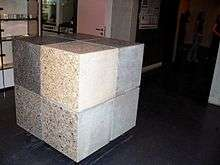# Cubic metreOne cubic metre of concrete (representing the world annual production per inhabitant)Look up cubic metre in Wiktionary, the free dictionary.

The cubic metre (in British English and international spelling as used by the International Bureau of Weights and Measures) or cubic meter (in American English) is the SI derived unit of volume. Its SI symbol is m3. It is the volume of a cube with edges one metre in length. An alternative name, which allowed a different usage with metric prefixes, was the stère, still sometimes used for dry measure (for instance, in reference to wood). Another alternative name, no longer widely used, was the kilolitre.

## Conversions

Main article: Unit conversion
 1 cubic metre = 1000 litres (exactly) ≈ 35.3 cubic feet ≈ 1.31 cubic yards ≈ 6.29 oil barrels ≈ 220 imperial gallons ≈ 264 US fluid gallons

A cubic metre of pure water at the temperature of maximum density (3.98 °C or 39.16 °F) and standard atmospheric pressure (101.325 kPa) has a mass of 1000 kg, or one tonne. At 0 °C (32 °F), the freezing point of water, a cubic metre of water has slightly less mass, 999.972 kilograms.

It is sometimes abbreviated to cu m, m3, M3, m^3, m**3, CBM, cbm when superscript characters or markup cannot be used (e.g. in some typewritten documents and postings in Usenet newsgroups).

Abbreviated CBM and cbm in the freight business and MTQ (or numeric code 49) in international trade.

## Multiples and submultiples

Main article: SI prefix

See Orders of magnitude (one cubic millimetre to one cubic metre) for a comparison with other volumes.

### Multiples

Cubic decametre
the volume of a cube of side length one decametre (10 m)
equal to a megalitre
1 dam3 = 1000 m3 = 1 ML
Cubic hectometre
the volume of a cube of side length one hectometre (100 m)
equal to a gigalitre
in civil engineering abbreviated MCM for million cubic metres
1 hm3 = 1000000 m3 = 1 GL
Cubic kilometre
the volume of a cube of side length one kilometre (1000 m)
equal to a teralitre
1 km3 = 1000000000 m3 = 1 TL

### Submultiples

Cubic decimetre also known as DCM in Rubber compound processing (Deci Cubic Meter).
the volume of a cube of side length one decimetre (0.1 m)
equal to a litre
1 dm3 = 0.001 m3 = 1 L
Cubic centimetre
the volume of a cube of side length one centimetre (0.01 m)
equal to a millilitre
1 cm3 = 0.000001 m3 = 10−6 m3 = 1 mL
Cubic millimetre
the volume of a cube of side length one millimetre (0.001 m)
equal to a microlitre
1 mm3 = 0.000000001 m3 = 10−9 m3 = 1 µL

## Notes

1. Bureau International de Poids et Mesures. "Derived units expressed in terms of base units". 2014. Accessed 7 August 2014.
2. From 1901 to 1964 the litre was defined as the volume of one kilogram of pure water at 4°C and 760 millimetres of mercury pressure. During this time, a litre was about 1.000028 dm3. In 1964 the original definition was reverted to.
3. "Archived copy". Archived from the original on 2009-10-01. Retrieved 2010-03-05.
4. The cubic centimetre is the base unit of volume of the CGS system of units. The colloquial abbreviations "cc" and "ccm" are not SI but are common in some contexts such as cooking, engine displacement and medicine.